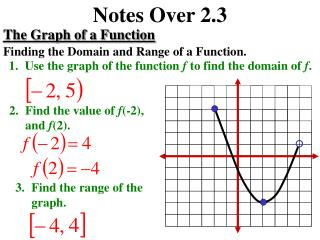Download PresentationNotes Over 2.3Notes Over 2.3 - PowerPoint PPT Presentation

Download PresentationNotes Over 2.3
An Image/Link below is provided (as is) to download presentation

Download Policy: Content on the Website is provided to you AS IS for your information and personal use and may not be sold / licensed / shared on other websites without getting consent from its author. While downloading, if for some reason you are not able to download a presentation, the publisher may have deleted the file from their server.

- - - - - - - - - - - - - - - - - - - - - - - - - - - E N D - - - - - - - - - - - - - - - - - - - - - - - - - - -
Presentation Transcript

1. Notes Over 2.3 The Graph of a Function Finding the Domain and Range of a Function. • Use the graph of the function f to find the domain of f. • Find the value of f(-2), and f(2). • Find the range of the graph.

2. Notes Over 2.3 Zeros of a Function Finding Zeros of a Function. Find the zeros of each function.

3. Notes Over 2.3 Zeros of a Function Finding Zeros of a Function. Find the zeros of each function.

4. Notes Over 2.3 Zeros of a Function Finding Zeros of a Function. Find the zeros of each function.

5. Notes Over 2.3 Increasing and Decreasing Functions Describe the increasing and decreasing behavior. The function is decreasing over the entire real line.

6. Notes Over 2.3 Increasing and Decreasing Functions Describe the increasing and decreasing behavior. The function is decreasing on the interval increasing on the interval decreasing on the interval increasing on the interval

7. Notes Over 2.3 Increasing and Decreasing Functions Describe the increasing and decreasing behavior. The function is increasing on the interval constant on the interval decreasing on the interval

8. Notes Over 2.3 Increasing and Decreasing Functions Determining Relative Maximum or Minimum. Relative Maximum Relative Minimum

9. Notes Over 2.3 Increasing and Decreasing Functions Determining Relative Maximum or Minimum. • Use a graphing calculator to find the relative maximum of the function:

10. Notes Over 2.3 Linear Functions 11. Write the linear function f for which and

11. Notes Over 2.3 Graphing a Piecewise Function Graph the function.

12. Notes Over 2.3 Even and Odd Functions Determine if the function is even, odd, or neither. Even function

13. Notes Over 2.3 Even and Odd Functions Determine if the function is even, odd, or neither. Odd function

14. Notes Over 2.3